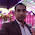We are already familiar with one-dimensional arrays or simple arrays. In one-dimensional arrays data stores in linear form or we consider it that data is stored in linear form. In one-dimensional arrays, there is only one subscript to access values in an array.

But we can also define two-dimensional arrays having two subscripts so also known as the double-subscripted arrays.

We can declare 2-D arrays just as we declare any simple array.

In one-dimensional arrays when we declare an array like
int arr;
This form 3 blocks in memory to store integer numbers.

But when we declare a 2-D array just as
int arr;
This will form 9 blocks in memory to store integer numbers.

You can relate this with a matrix in calculus with three rows and three columns or 3-by-3 matrix.

The first subscript shows the total number of rows and second subscript shows the total number of columns.

You can understand by understating this image
As you know index in arrays start from 0. So the row numbers are 0,1,2 and column numbers are also 0,1,2.

We can access any block by writing the name of the 2-D array and then giving row subscript first and then column subscript.

If I want to access the block with row number 1 and column number also 2. I will write arr.

If we want to initialize it we can initialize it as
arr=1;
arr=2;
arr=3;
arr=4;
arr=5;
arr=6;
arr=7;
arr=8;
arr=9;

After this initialization, the array will have values in it just as shown
We can also initialize two-dimensional arrays like
int b[ 2 ][ 2 ] = { { 1, 2 }, { 3, 4 } };
This will store integers in memory just as
If we miss any number from the list of data items just as we missed 2 from the data the array will automatically save 0 in their location.
For example, if we write
int b[ 2 ][ 2 ] = { { 1 }, { 3, 4 } };
this will save values just as
You can see at b there is a 0 which is automatically assigned.

Program to get values from the user in the 2-D array:
#include<stdio.h>
int main(){

int arr,i,j;

printf("Enter values in Two Dimensional array:\n\n");
for(i=0;i<2;i++){
for(j=0;j<3;j++){
printf("arr[%d][%d]: ",i,j);
scanf("%d",&arr[i][j]);
}
}

printf("\n\nValues in Two Dimensional arrays are:\n\n");
for(i=0;i<2;i++){
for(j=0;j<3;j++){
printf("%d ",arr[i][j]);
}
printf("\n");
}

return 0;
}

Output:

Enter values in Two Dimensional array:

arr: 5
arr: 3
arr: 2
arr: 7
arr: 4
arr: 3

Values in Two Dimensional arrays are:

5 3 2

7 4 3

In the program, I have declared a two-dimensional array with 2 rows and 3 columns. As 2*3=6 So I can save 6 values in that array.
I have used two loops the first loop is for rows and second is for columns both starts from zero and ends at size-1.
When the first loop execute the value of i (row) will be 0 and in the second loop the value of i (row) will remain the same and by increasing the value of j (column) we can access each block with zero row and save values in it. Same will happen when i equal to 1.

1.2.